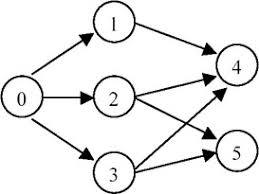I am sure some of you might have heard about topological sorting, but do you really know what that is? Let's find out together!

From the Wikipedia's definition, a topological sort of a directed graph is a linear ordering of its vertices such that for every directed edge `uv` from vertex `u` to vertex `v`, `u` comes before `v` in the ordering.

In layman's term, a topological sorting is to sort a directed graph that has no cycles. Normally, you will use an array list or any other data structures to store the result of the sorting. The way we sort the graph is by calculating the indegree of each node, which is the number of neighbor nodes pointing to itself.

NOTE: topological sorting of a graph cannot be accomplished if the graph has formed cycles between nodes. A topological ordering is possible `iff` the graph has no directed cycles, that is, it it is a directed acyclic graph (`DAG`).

There are quite a lot of good articles regarding to topological sort, so I will skip the remaining explanation part here. Here's one that I find quite useful, the link is attached here for reference.

### Given an directed graph, find any topological order for the given graph.

You can think of the node without other nodes pointing to it as the initial node.The topological order can be `[0,1,2,3,4,5]` or `0,2,3,1,5,4]` and etc.

Here's the solution for reference.

``````    public ArrayList<DirectedGraphNode> topSort(ArrayList<DirectedGraphNode> graph) {

ArrayList<DirectedGraphNode> order = new ArrayList<>();
if(graph == null){
return order;
}

// 1. we need to find out the indegree for each node
Map<DirectedGraphNode, Integer> indegree = getIndegree(graph);

// 2. add the node with 0 indegree into the queue and array
for(DirectedGraphNode node : graph){
if(indegree.get(node) == 0){
queue.offer(node);
}
}

// 3. decrease the indegree level after removing the 0 degree node
// add the node with 0 indegree to queue and array
while( !queue.isEmpty()) {
DirectedGraphNode node = queue.poll();
for(DirectedGraphNode neighbor : node.neighbors){
indegree.put(neighbor, indegree.get(neighbor) - 1);
if(indegree.get(neighbor) == 0){
queue.offer(neighbor);
}
}
}

// 4. check if the sorted array's size is the same as initial graph size
if(order.size() == graph.size()){
return order;
}

return null;
}

private Map<DirectedGraphNode, Integer> getIndegree(ArrayList<DirectedGraphNode> graph){

Map<DirectedGraphNode, Integer> indegree = new HashMap<>();

// 1. initialize the map
for(DirectedGraphNode node : graph){
indegree.put(node, 0);
}

// 2. add the indegree level for each pointed node
for(DirectedGraphNode node : graph){
for(DirectedGraphNode neighbor : node.neighbors){
indegree.put(neighbor, indegree.get(neighbor) + 1);
}
}
return indegree;
}
}
``````

The solution is actually pretty neat and straightforward. For `BFS` problem, the procedure is almost the same with little modification. For starter, you will need a hashmap or array and a queue. While the queue is not empty, you remove the current node from the queue and add the neighbor nodes into the queue. When certain criteria is met, for example, in this particular question, we add the neighbor node into the queue when the indegree of the node becomes 0.

That's all about it! It ain't that hard, right? I will be posting related `BFS` problems in the next few posts, so stay tuned!

Remember, the more you practice, the better you get! Let's do it 😃

Post was published on , last updated on .

Like the content? Support the author by paypal.me!